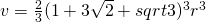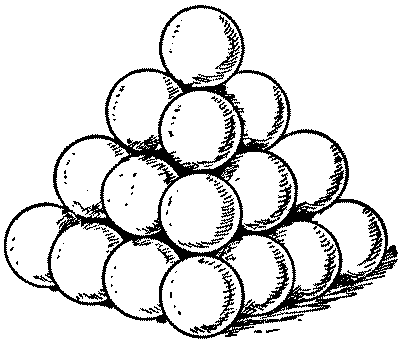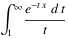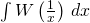## Programming challenge: Which words have the most anagrams?

September 18th, 2013

While on the train to work this morning, I wondered which English words have the most anagrams that are also valid English words. So, I knocked up few lines of Mathematica code and came up with 4 sets of 7:

{{"ates", "east", "eats", "etas", "sate", "seat", "teas"},
{"pares", "parse", "pears", "rapes", "reaps", "spare", "spear"},
{"capers", "crapes", "pacers", "parsec", "recaps", "scrape", "spacer"},
{"carets", "caster", "caters", "crates", "reacts", "recast", "traces"}}

So, according to my program (and Mathematica’s dictionary), the best you can do is 7.  I’m not going to post the code until later because I don’t want to influence the challenge which is ‘Write a program in your language of choice that queries a dictionary to find which English words have the most anagrams that are also valid English words.’

## Probability of overlapping sub-sequences?

October 8th, 2011

Consider a periodic sequence, seqp, with period p

seqp = a1, a2, …, ap,  a1, a2, …, ap,  a1, a2, …, ap, …

Two sub-sequences, each of length N where 2< N< p, are produced by choosing two random start points in seqp and using the next N-1 numbers. What is the probability that these two sub-sequences will overlap?

If you take M>2 such length N sub-sequences then what is the probability that any two will overlap?

For a more concrete example, assume that p = 2^19937 – 1 (yes, a very big number) and consider 10,000 sub-sequences each of length 10^9.

Disclaimer: I don’t have the solution to this and haven’t yet tried to find it

## RationalQ – Testing for rationals in Mathematica

August 30th, 2009

Mathematica has a large set of functions that you can use to test the properties of numbers.  For example

IntegerQ[x]

returns True if x is an integer and False if it isn’t.  Of course you are not just restricted to asking if x is an integer or not.  For example, you can ask if it is an even number

EvenQ[x]

or an odd number

OddQ[x]

Perhaps you are wondering if x is prime

PrimeQ[x]

or even if it is an algebraic integer

AlgebraicIntegerQ[x]

The observant reader will notice that all of these functions end with a capital Q and a way of remembering this is to think that you are asking a question of the variable x.  So the question ‘Is x an integer‘ becomes, in Mathematica notation, IntegerQ[x].

I am currently working on a piece of code where I need to determine whether or not a particular number belongs to the set of rationals and I assumed that a suitable function would exist in Mathematica and that it would be called RationalQ[] so I was rather surprised to see that there is no such function in Mathematica 7.

So, I’ll just have to come up with my own.  A quick search resulted in the following function definition from Bob Hanlon

Which almost does what I need.  It handles the following correctly

RationalQ[1/2] (gives True)

RationalQ[Sqrt] (Gives False)

but I needed a version of RationalQ that also returned True when passed an integer.  After all, the integers are just a subset of the rationals.  A moments thought resulted in

RationalQ[x_] := (Head[x] === Rational || IntegerQ[x]);

Which seems to work perfectly.  So, I offer the above function for anyone who is googling for a RationalQ function and I also ask the following questions to any Mathematica gurus who might be reading this

• Is there anything wrong with the above definition?
• Why isn’t such an obvious function not included in Mathematica as standard?

## Proof that math exams have become easier over the last 169 years!

June 22nd, 2009

Solution to problem of the week #6

Way back in April, I posed the following problem.  ‘Consider a square pyramidal pile of identical cannonballs of radius r such that the bottom layer contains 16 cannonballs (such as the pile in the diagram above). Find the volume (in terms of r) of the pyramid that envelops and contains the whole pile’

Since then I have received several answers (check out the comments section of the original post for a few of them) and all but one of them were wrong.  In my opinion, this is nothing to be too ashamed about since I couldn’t solve the problem either and I am not about to berate my readers for failing to do something that I couldn’t do myself!

So if I couldn’t do it then how did I know that all of these answers were incorrect?  Well clearly I had cheated and had access to a worked solution.  ‘My’ problem was in fact problem number 15 from one the 1840 undergraduate final exams at Cambridge University and a worked solution is given in the (now fully digitized, thanks to google) text Solutions for the Cambridge Problems 1840,1841.

The solution isYou’ll find the official worked solution to this problem on page 20 of ‘Solutions for the Cambridge Problems 180,1841’ (sadly, the diagram is missing) but James Graham-Eagle of the University of Massachusetts Lowell sent me not one but two different solutions in this pdf file and they are much easier to follow in my humble opinion.  Thanks for that James.

I’m glad that this problem wasn’t in my final exam!

## Problem of the week #6 – Cannonballs

April 17th, 2009

Imagine that you were the Captain of a sailing ship a few hundred years ago and, in order to protect yourself from pirates, you had a few cannons.  Cannons need cannonballs and it is well known that the best way to stack cannon balls is to arrange them as a square pyamid as in the image below.So, in this example you have 16 balls in the bottom layer, 9 balls in the next layer, then 4 and, finally, one at the top giving a total of 16+9+4+1 = 30 balls and we say that 30 is the 4th square pyramidal number.  The first few such numbers are

• 1
• 5 (4+1)
• 14 (9+4+1)
• 30 (16+9+4+1)
• 55 (25+16+9+4+1)

Now, there is a well known problem called the Cannonball problem (Spolier alert: This link contains the solution) which asks ‘What is the smallest square number that is also square pyramidal number?’ but the traditional cannonball problem has been stated and solved by many people and so it isn’t my problem of the week.

My problem is as follows ‘Consider a square pyramidal pile of identical cannonballs of radius r such that the bottom layer contains 16 cannonballs (such as the pile in the diagram above). Find the volume (in terms of r) of the pyramid that envelops and contains the whole pile

As always, there are no prizes I’m afraid (but if you are a company who would like to sponsor prizes for future POTWs then let me know).  I imagine that the solution to this will require a diagram so it might be best to put your solution on a pdf file, web page or some other visual media rather than using the comments section.  Finding my email adress is yet another (easy) puzzle to solve.

Have fun.

## Solution to problem of the week #3 (and an apology)

April 8th, 2009

From time to time I post a problem of the week on Walking Randomly and invite readers to post solutions.  Although I haven’t done many, they seem to be quite popular and so I plan on resurrecting the series soon.

Back in August last year I posted a problem concerning Fibonacci numbers and matrices and Sam Shah (Of Continuous Everywhere but Differentiable Nowhere fame) took the time to write out a full solution in a PDF file and sent it to me via email.  I promised that I would publish it ‘soon’.

Well…umm…’soon’ turned out to be almost a year later.  Here is Sam’s solution to the original problem – enjoy!

Sorry Sam!

## Solution to Integral of the Week #5

January 22nd, 2009

Near the beginning of the month I posted a new Integral of the week which asked for the indefinite integral of

W(1/x)

Where W is the Lambert W function.  I had a response from Simon Tyler who sent me a PDF file with a full solution and as far as I can tell it is correct but feel free to get in touch if you disagree with us.

In case you don’t want to read the PDF file, the solution is

x*W(1/x) + E1(W(1/z))

Where E1 stands for the Exponential Integral which is defined asIn Mathematica notation this solution is written as

x*ProductLog[1/x] + ExpIntegralE[1, ProductLog[1/x]]

Since Mathematica uses the function name ProductLog to stand for the Lambert W function.  Let’s differentiate this to see if it is correct

D[x*ProductLog[1/x] + ExpIntegralE[1, ProductLog[1/x]], x]

gives ProductLog[1/x]

So, why did I select this particular integral as integral of the week you may ask?  Well it started off with a thread in the Maxima developers mailing list.  The developers of Maxima added the Lambert W function fairly recently and the system was having serious problems finding the integral W(1/x).  If you evaluate

integrate(lambert_w(1/x),x)

in Maxima 5.17.1 then the integrator just loops endlessly – never finding a solution. The members of the maxima developers forum knew what the result should be but they couldn’t get maxima to find it. This got me curious so I fired up Mathematica 7 and did

Integrate[ProductLog[1/x],x]

Mathematica replied by simply returning the unevaluated integral to me. So, not only did Maxima fail at this particular integral but so did Mathematica. Could MATLAB 2008b do any better with its new Mupad-based symbolic toolbox?
 syms x int(lambertw(0, 1/x), x)

Warning: Explicit integral could not be found.
> In sym.int at 64

That’ll be a no then! I can’t try Maple 12 since I don’t have a copy but I do have a copy of Matlab 2007b which uses the Maple 10 kernel as part of its symbolic toolbox so how did that do?
 syms x int(lambertw(0, 1/x), x)

ans=x*lambertw(1/x)+Ei(1,lambertw(1/x))

Success! So Maple (via MATLAB 2007b) is the winner in this little game it seems – I really need to get myself a full copy of the thing.

So, since 3 out of 4 powerful computer algebra systems couldn’t do this integral, I wondered if maybe I could. Turns out that I couldn’t! It seems my interest in bizarre looking integrals far outstrips my ability to actually do them. So, I threw it open to you guys and, thankfully, Simon rose to the challenge. Thanks again Simon.

## Integral of the week #5

January 3rd, 2009

I’ve not done one of these for a while. Calculate the following indefinite integral.Where the W stands for the Lambert W function.

Full disclosure: I know the result and have verified it by differentiating but I don’t know how to obtain it.

Update: The solution has been posted here.

## Problem of the week #4

October 24th, 2008

I haven’t done a ‘problem of the week’ for a while so I thought I would throw a fun one out there to see what happens. Prove (or otherwise) that 0.9 recurring (that is 0.999999999999…… etc) is equal to one.

Update: Several solutions have been posted in the comments section

## Problem of the week #3

August 1st, 2008

No integral this time – I’m saving those for another day. This week’s problem is

Give a proof of the relationship between the Fibonacci Numbers and the determinant of the matrices discussed in this post.

As always, this is just for fun as there are no prizes (unless, of course, some kind sponsor would like to help me out).

Feel free to give submissions via the comments section but, be warned, it doesn’t support latex. Probably the best way to submit your solutions would be to send them to my email address. I’ll take just about any format – even Microsoft word – but pdf or Latex is probably best. Solutions that I think to be correct will be published here at a later date (subject to obtaining your permission of course).

Have fun.